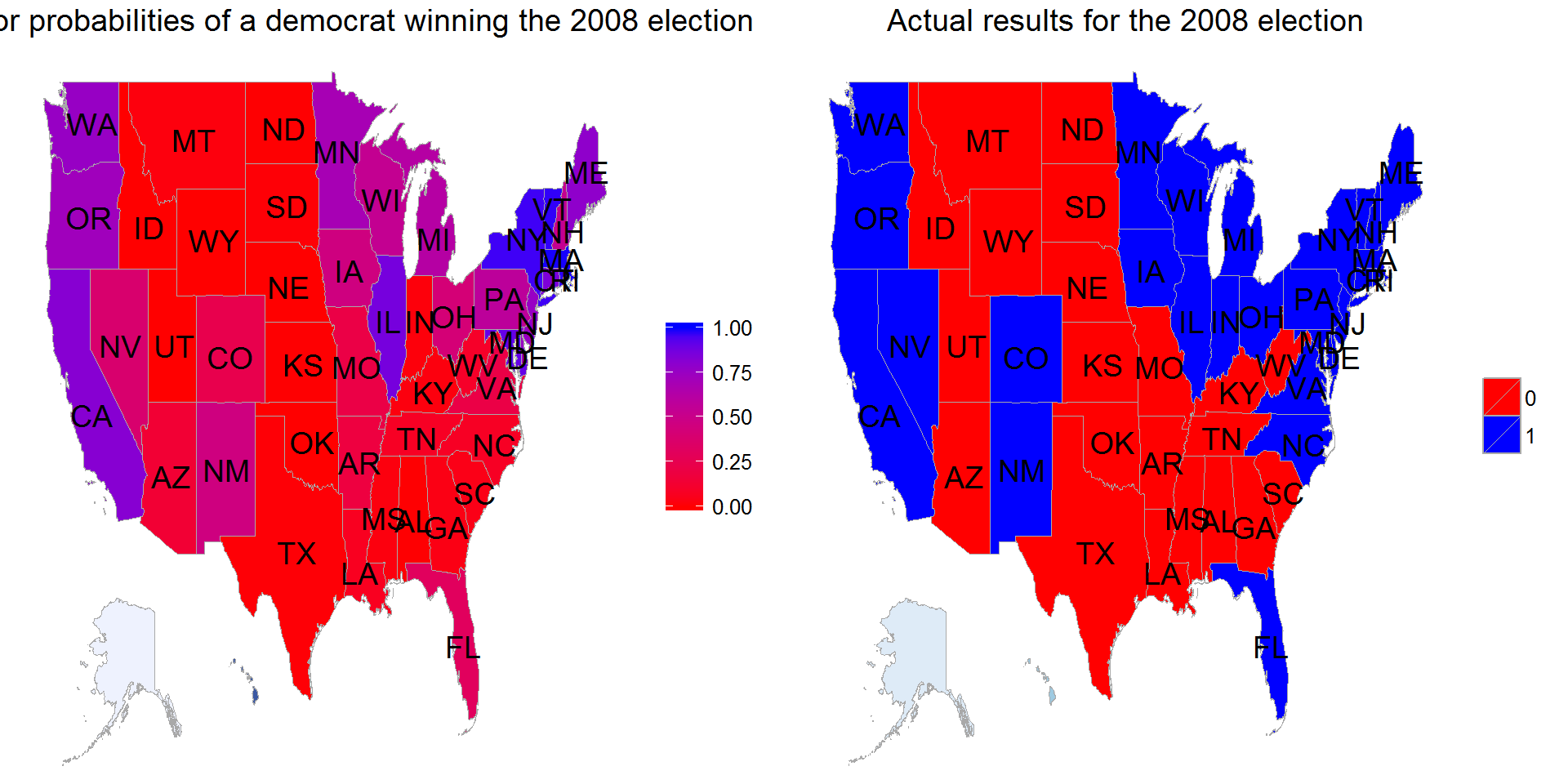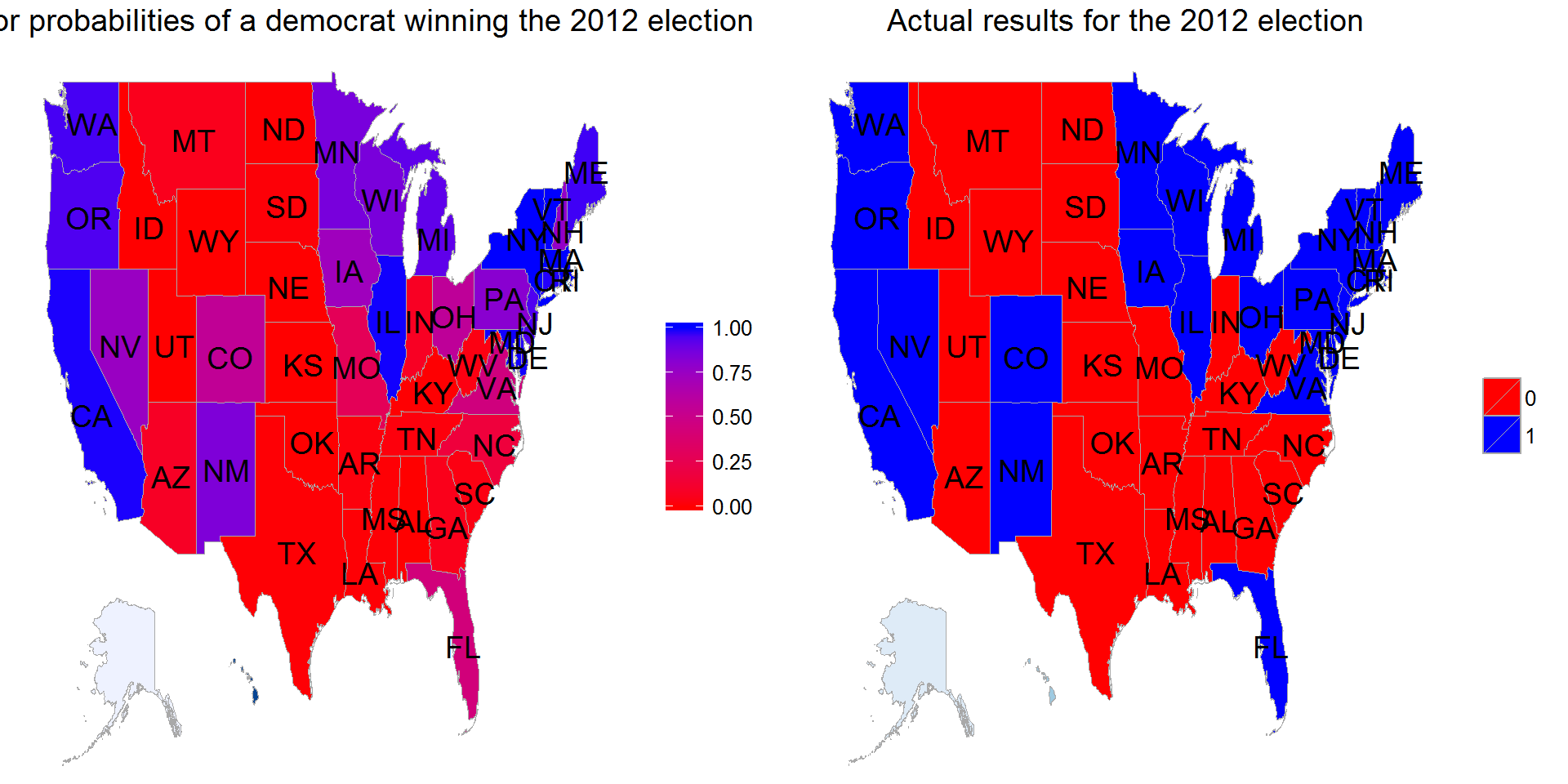``````> library(car)
> library(caret)
> library(ggplot2)
> library(reshape2)
> library(gridExtra)
> library(choroplethr)
> library(choroplethrMaps)``````

# Bayesian approach to predicting the US elections.

``> data <- read.csv("final_data.csv")``
``````> df = data.frame(region=tolower(data\$state), value=data\$winner_2008)
> map_2008 <- state_choropleth(df)
> map_2008 <- map_2008 + scale_fill_manual(name=" ", values=c("red", "blue"))
> map_2008 <- map_2008 + ggtitle("Actual results for the 2008 election")
>
> df = data.frame(region=tolower(data\$state), value=data\$winner_2012)
> map_2012 <- state_choropleth(df)
> map_2012 <- map_2012 + scale_fill_manual(name=" ", values=c("red", "blue"))
> map_2012 <- map_2012 + ggtitle("Actual results for the 2012 election")``````

A function to simulate `n` elections. Input a list of state win probabilities `probabilities` and number of simulations `n`. The result is a binomial random variable with the given probability of 1 i.e democrat win.

``````> simulate_election <- function(probabilities, n){
+     results <- numeric(n)
+     for(i in 1:n){
+         for(j in 1:nrow(data)){
+             state <- data\$state[j]
+             if(rbinom(1, 1, probabilities[j]) == 1){
+             }
+         }
+     }
+     results
+ }``````

Use an alternate Bayesian approach to predicting the 2012 election results, post-facto, as a way to develop a model for predicting future election results. Start with a flat prior distribution for each state.

``````> states <- data\$state
> alpha0 <- rep(1, 51)
> beta0 <- rep(1, 51)``````

Update using 2004 data to obtain posterior distribution. We use percentage of democrats in each state.

``````> alpha1 <- alpha0 + data\$democrat_04
> beta1  <- beta0 + data\$republican_04``````

Calculate the state win probabilities for democrats in 2008 `prob_win_08`

``````> n <- nrow(data)
> prob_win_08 <- numeric(n)
> for (i in 1:n) {
+     prob_win_08[i] <- round(1 - pbeta(0.5, alpha1[i], beta1[i]), digits=3)
+ }``````

Predict a democratic win in 2008 for a state if the computed probability of a win is >= 0.50

``````> states <- data\$state
> pred_democ_win_08 <- states[prob_win_08 >= 0.50]
> actual_democ_win_08 <- data[data\$winner_2008 == 1,]\$state
> setdiff(actual_democ_win_08, pred_democ_win_08)``````
``````##  "Colorado"       "Florida"        "Indiana"        "Iowa"
##  "Nevada"         "New Mexico"     "North Carolina" "Ohio"
##  "Virginia"``````

The prediction misses the following 9 states: `Colorado`, `Florida`, `Indiana`, `Iowa`, `Nevada`, `New Mexico`, `North Carolina`, `Ohio` and `Virginia`.

Compare posterior probabilities of a democrat winning the 2008 election with the actual results of the 2008 election.

``````> df = data.frame(region=tolower(data\$state), value=prob_win_08)
> map <- state_choropleth(df, num_colors=1)
> map <- map + scale_fill_continuous(name="", low="red", high="blue")
> map <- map + ggtitle("Posterior probabilities of a democrat winning the 2008 election")
>
> grid.arrange(map, map_2008, ncol=2)``````Update using 2008 data to obtain posterior distribution. We use percentage of democrats in each state.

``````> alpha2 <- alpha1 + data\$democrat_08
> beta2  <- beta1 + data\$republican_08``````

Calculate the state win probabilities for democrats in 2012 `prob_win_12`

``````> prob_win_12 <- numeric(n)
> for (i in 1:n) {
+     prob_win_12[i] <- round(1 - pbeta(0.5, alpha2[i], beta2[i]), digits=3)
+ }``````

Predict a democratic win in 2012 for a state if the computed probability of a win is >= 0.50

``````> pred_democ_win_12 <- states[prob_win_12 >= 0.5]
> actual_democ_win_12 <- data[data\$winner_2012 == 1,]\$state
> setdiff(actual_democ_win_12, pred_democ_win_12)``````
``##  "Florida"  "Virginia"``

The difference between predicted wins and actual is far narrower this time with misses on the following states: `Florida` and `Virginia`.

We compare posterior probabilities of a democrat winning the 2012 election with the actual results of the 2012 election.

``````> df = data.frame(region=tolower(data\$state), value=prob_win_12)
> map <- state_choropleth(df, num_colors=1)
> map <- map + scale_fill_continuous(name="", low="red", high="blue")
> map <- map + ggtitle("Posterior probabilities of a democrat winning the 2012 election")
>
> grid.arrange(map, map_2012, ncol=2)``````Update using gallup 2012 data to obtain posterior distribution. We use gallup sampling results before the 2012 elections in each state.

``````> alpha3 <- alpha2 + data\$gallup_democrat_2012
> beta3  <- beta2 + data\$gallup_republican_2012``````

Calculate the probability of a democratic win in 2012 based on this posterior distribution for each state.

``````> rev_prob_win_12 <- numeric(n)
> for (i in 1:n) {
+     rev_prob_win_12[i] <- round(1 - pbeta(0.5, alpha3[i], beta3[i]), digits=3)
+ }``````

Predict a democratic win in 2012 for a state if the computed revised probability of a win is >= 0.50

``````> rev_pred_democ_win_12 <- states[rev_prob_win_12 >= 0.5]
> actual_democ_win_12 <- data[data\$winner_2012 == 1,]\$state
> setdiff(actual_democ_win_12, rev_pred_democ_win_12)``````
``##  "Colorado" "Florida"  "Virginia"``

The Gallup data based revision includes `Colorado` among the states where prediction differs from the actual outcome.

Compare posterior probabilities of a democrat winning the 2012 election with the actual results of the 2012 election.

``````> df = data.frame(region=tolower(data\$state), value=rev_prob_win_12)
> map <- state_choropleth(df, num_colors=1)
> map <- map + scale_fill_continuous(name="", low="red", high="blue")
> map <- map + ggtitle("Revised posterior probabilities of a democrat winning the 2012 election")
>
> grid.arrange(map, map_2012, ncol=2)``````